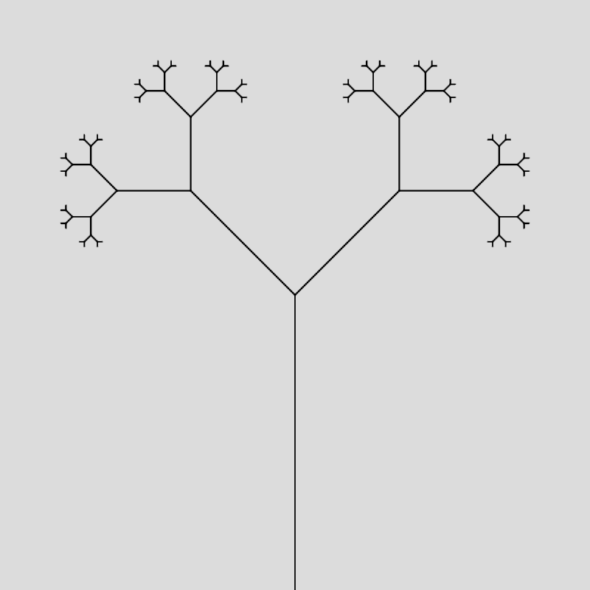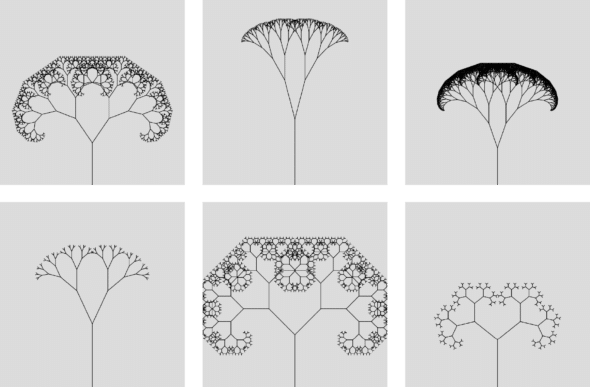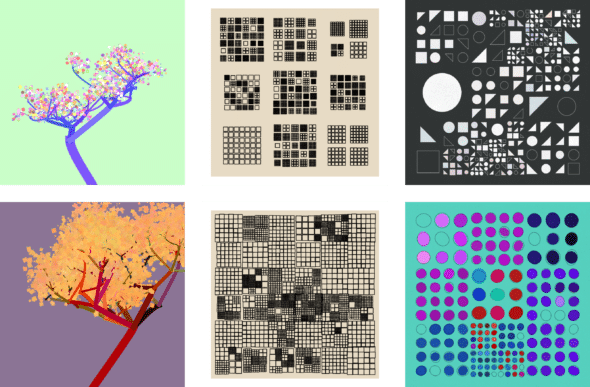# Generative Art: Dive Deeper With Recursion

### Article summary

In my previous post on generative art, we used Python’s Turtle library to learn the basics of generative art. In this post, we’ll dive deeper and create a generative art program that uses recursion to produce infinitely complex pieces. This time, we’ll be using p5.js.

## Why use recursion?

You might remember recursion from your computer science courses in college and think, “How does this apply to art?” It may seem like a rigid, mathematical concept, but as you can see in the examples below, recursion manifests itself in the natural world. These patterns repeat upon themselves in a beautiful, unique way, and we can use these patterns to inspire our art.

## Let’s dive in.

To explore recursion in generative art, we’re going to build a program that draws a tree. Trees, as you know, are inherently recursive data structures used in many computer algorithms, but they’re also naturally-occurring lifeforms with bark and leaves that grow out of the ground. We’ll combine both of our tree definitions in our tree-drawing program.

First off, open up the p5.js web editor. You’ll notice that the project is initialized with some boilerplate code, which sets up a blank canvas with a gray background.

``````
function setup() {
createCanvas(400, 400);
}

function draw() {
background(220);
}
``````

The one modification we’ll make to this boilerplate is adding a call to `noLoop` inside of `setup`. By default, p5.js re-runs `draw` on every frame. This is useful for animated pieces, but since we’re drawing a static image, we can use `noLoop` to disable this behavior.

``````
function setup() {
createCanvas(400, 400);
noLoop();
}
``````

Next, let’s write the signature for our recursive tree function:

``````
function drawTree(x, y, angle, length) {}
``````

In our function, `x` and `y` will represent the coordinates of the base of the tree, `angle` will denote the angle in radians at which the tree is leaning, and `length` will denote the length, in pixels, of the tree’s trunk.

Let’s continue with a non-recursive implementation of our function:

``````
function drawTree(x, y, angle, length) {
const [x1, y1] = [x, y];
const x2 = x1 + cos(angle) * length;
const y2 = y1 - sin(angle) * length;

line(x1, y1, x2, y2);
}
``````

Using a bit of trigonometry, this function draws a line starting at (`x`, `y`) with an `angle` and `length` as specified in the parameters. Note that in p5.js, the origin of the coordinate plane is the top-left corner, so y-coordinates increase in value as you go down the canvas, hence the `-` in the `y2` calculation.

Try calling the `drawTree` function inside of `draw` to verify that it works as expected. This invocation, for example, should draw a line starting at the bottom-center of the screen at a 90-degree angle and at a length of 1/2 of the canvas:

``````
drawTree(
width / 2,
height,
PI / 2,
height / 2
);
``````

Now let’s add the recursive step to our function. We’ll branch into two sub-trees, one leaning left and one leaning right, each half the length of the previous tree. Of course, since this is a recursive function, we also need to define a base case. We’ll set a `minLength` of 5 at the top of our program, and stop recursing if `length` falls below it.

``````
const minLength = 5;

function drawTree(x, y, angle, length) {
const [x1, y1] = [x, y];
const x2 = x1 + cos(angle) * length;
const y2 = y1 - sin(angle) * length;

line(x1, y1, x2, y2);

if (length >= minLength) {
drawTree(x2, y2, angle + PI / 4, length / 2);
drawTree(x2, y2, angle - PI / 4, length / 2);
}
}
``````

With this modification, we get our first tree-like output:Right now, our program produces the exact same output on every run, which is a little boring. Let’s fix that by moving our inputs to variables and giving them random values in the `setup` function.

``````
let baseLength;
let minLength;
let lengthRatio;
let angleChange;

function setup() {
createCanvas(400, 400);
noLoop();

baseLength = random(height / 8, height / 2);
minLength = random(1, 10);
lengthRatio = random(0.25, 0.75);
angleChange = random(PI / 32, PI / 3);
}

function draw() {
background(220);

drawTree(width / 2, height, PI / 2, baseLength);
}

function drawTree(x, y, angle, length) {
const [x1, y1] = [x, y];
const x2 = x1 + cos(angle) * length;
const y2 = y1 - sin(angle) * length;

line(x1, y1, x2, y2);

if (length >= minLength) {
drawTree(x2, y2, angle + angleChange, length * lengthRatio);
drawTree(x2, y2, angle - angleChange, length * lengthRatio);
}
}
``````

Re-running our program now, we get results that look much more diverse:Of course, I promised we were going to take inspiration from real-life trees here, so let’s add some leaves. We’ll add a `drawLeaves` function with the following signature:

``````
function drawLeaves(x, y) {}
``````

Next, we’ll add a couple more variables to the top of our file, `leafDensity` and `leafColor`, and randomize them in `setup`.

``````
let leafDensity;
let leafColor;

// in setup
leafDensity = random(0, 10);
leafColor = color(
random(0, 255),
random(0, 255),
random(0, 255)
);
``````

Finally, we’ll implement our `drawLeaves` function and call it in the `else` clause of our `drawTree` function, so leaves are only drawn at the end of branches.

``````
function drawTree(x, y, angle, length) {
const [x1, y1] = [x, y];
const x2 = x1 + cos(angle) * length;
const y2 = y1 - sin(angle) * length;

line(x1, y1, x2, y2);

if (length >= minLength) {
drawTree(x2, y2, angle + angleChange, length * lengthRatio);
drawTree(x2, y2, angle - angleChange, length * lengthRatio);
} else {
drawLeaves(x2, y2);
}
}

function drawLeaves(x, y) {
push();

fill(leafColor);
noStroke();

for (let i = 0; i < leafDensity; i++) {
circle(
randomGaussian(x, 10),
randomGaussian(y, 10),
random(2, 5)
);
}

pop();
}
``````

• The `push` and `pop` functions allow us scope styles like fill and stroke to this particular function call. This allows us to apply `noStroke` to the leaves while still using the default stroke on the branches.
• `randomGaussian` produces random numbers using a normal (bell-shaped) distribution, unlike `random` which uses a uniform distribution. This allows us to make the leaves cluster close to (`x`, `y`). Normal distributions are found more commonly in nature than uniform distributions, so `randomGaussian` is usually the way to go when you want to mimic nature.

When we run the program now, we get the following results, and with that, our program is done!## It doesn't stop there

Recursion allows us to generate beautiful, complex pieces with just a few lines of code. This tree example only scratches the surface. Below are some of my recent pieces using recursion, to give a few examples of the possibilities:What will you create?

Conversation